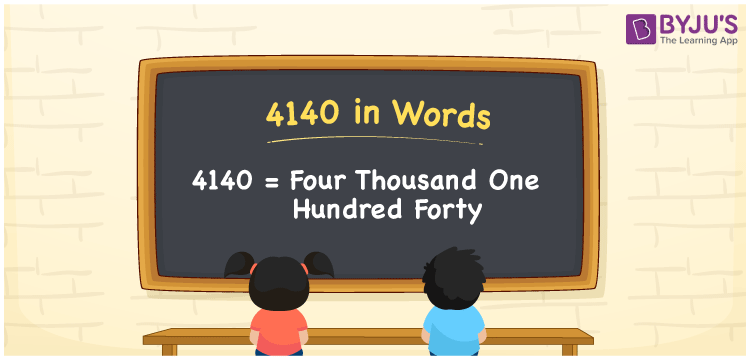# 4140 in Words

4140 in words is written as Four thousand one hundred forty. In both the International System of Numerals and the Indian System of Numerals, 4140 is written as Four thousand one hundred forty. The number 4140 is a Cardinal Number as it could represent some quantity. For example, “the area of this land is 4140 metres square”.

 4140 in Words Four thousand one hundred forty Four thousand one hundred forty in Number 4140

## 4140 in English Words

4140 in English words is read as “Four thousand one hundred forty”.## How to Write 4140 in Words?

To write 4140 in words, we shall use the place value chart. In the place value chart, put 4 in the thousands, 1 in the hundreds, 4 in the tens, and 0 in the ones, respectively. Let us make a place value chart to write the number 4140 in words.

 Thousands Hundreds Tens Ones 4 1 4 0

Thus, we can write the expanded form as

4 × Thousand + 1 × Hundred + 4 × Ten + 0 × One

= 4 × 1000 + 1 × 100 + 4 × 10 + 0 × 1

= 4000 + 100 + 40 + 0

= 4140

= Four thousand one hundred forty.

4140 is a natural number which is the successor of 4139 and the predecessor of 4141.

4140 in words – Four thousand one hundred forty

• Is 4140 an odd number? – No
• Is 4140 an even number? – Yes
• Is 4140 a perfect square number? – No
• Is 4140 a perfect cube number? – No
• Is 4140 a prime number? – No
• Is 4140 a composite number? – Yes

## Frequently Asked Questions on 4140 in Words

Q1

### How to write 4140 in words?

4140 in words is written as Four thousand one hundred forty.
Q2

### How to write 4140 in the International and Indian System of Numerals?

In both, the system of numerals, 4140 in words, is written as Four thousand one hundred forty.
Q3

### How to write 4140 in a place value chart?

In the place value chart, write 4 in the thousands, 1 in the hundreds, 4 in the tens, and 0 in the ones, respectively.